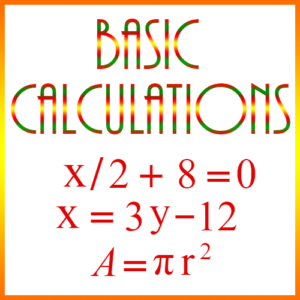#Basic Calculations

Basic Calculation problems are typically problems which can be solved with the simple application of a formula, possibly with an equation, or by carrying out “common sense” computations. This page has 4 examples. However, the first example has 4 versions. To practice more of these types of problems, click here.

Example 1)

A school district is ordering blackboard erasers for a new school. Each of the 16 classrooms needs 6 erasers. If a box contains 8 erasers, and a case contains 4 boxes, how many cases should be ordered?

A. 1
B. 2
C. 3
D. 4
E. 5

Explanation: This problem can be formulated as an equation. Let n be the number of cases the district needs to order. Then

16∙6 = 8∙4∙n
96 = 32n

So,

96/32 = 3 = n

Now let’s look at a second version of the same problem:

A school district is ordering blackboard erasers for a new school. Each of the 16 classrooms needs 6 erasers. A box contains 8 erasers, and a case contains b boxes. If 3 cases are ordered, what is b?

A. 1
B. 2
C. 3
D. 4
E. 5

Explanation: In this version, we are not given the number of boxes in a case, but we are given the number of cases that were ordered. So, this problem can be formulated by using a variation of the equation above:

16∙6 = 8∙b∙3
96 = 24b

So,

96/24 = 4 = b

Here is a third version of the same problem:

A school district is ordering blackboard erasers for a new school. Each of the 16 classrooms needs 6 erasers. A box contains e erasers, and a case contains 4 boxes. If 3 cases are ordered, what is e?

You should be able to see that you can solve this problem using the same formula we used for the solution to the other two versions, but substituting the appropriate variable and number in the appropriate place.

So now let’s make the problem more abstract for our 4th version. That is, suppose we have multiple variables in the problem. Here’s an example:

A school district is ordering blackboard erasers for a new school. Each of the m classrooms needs 6 erasers. If a box contains e erasers, and a case contains b boxes, how many cases should be ordered?

A. ebm/6
B. 6eb
C. 6meb
D. 6m/eb
E. eb/6m

Explanation: With this version of the problem we can still use the formula, but now we have to deal with several variables. Suppose the number of cases to be ordered is n. Then we have this relationship:

m∙6 = e∙b∙n
6m = ebn

So,

6m/eb = n

Example 2)

On an existing foundation Bill wants to build a wall 30 feet long, 6 feet high, and 4 inches thick with cinder blocks. Including the width of mortar between blocks, each block is 9 inches long, by 6 inches high, and 4 inches wide. Every second row of blocks will start and end with a half-block, which he will make by cutting an existing block. If the blocks cost $.80 each, what will be his cost for the blocks? A.$384
B. $360 C.$404
D. $334 E.$484

Explanation: Obviously, we need to figure out how many blocks he will need. So if each row is 30 feet long, then the number of inches in a row is

30 × 12 = 360

And if each block is 9 inches long, then the number of blocks per row is

360/9 = 40

Now we need to determine how many rows there will be. Since the wall is 6 feet high, and each block is 6 inches high, then the number of rows is

(6 × 12)/6 = 12

Thus, 12 rows at 40 per row yields 480 blocks.

Each block costs $.80, so the total cost of blocks is .80 × 480 =$384

So the correct answer is A.

Example 3)

Acme Limousine Service operates a fleet of 50 vehicles. Suppose each car is driven 24,000 miles in a year. If the 30 model N cars used 36,000 gallons of gas in the year, and the 20 model M cars used 32,000 gallons, what is the difference in the miles per gallon averages of the two sets of cars?

A. 3 miles per gallon
B. 8 miles per gallon
C. 5 miles per gallon
D. 10 miles per gallon
E. 2 miles per gallon

Explanation: On the average the Model N and Model M cars used this many gallons of gas each:

Model N gallons = 36,000/30 = 1200
Model M gallons = 32,000/20 = 1600

Thus,

Model N miles per gallon = 24,000/1200 = 20
Model M miles per gallon = 24,000/1600 = 15

Therefore, the difference between the two is 20 – 15 = 5

Example 4)

An electronics store got a shipment of 50 computers for \$200 each, and marked them up 30% for sale. After 40 were sold, they reduced the price by 50%, and sold the remainder. Based solely on the store’s purchase cost of the computers, what was the percentage of their profit or loss?

A. Profit of 17%
B. Loss of 10%
C. Profit of 21%
D. Loss of 12%
E. Profit of 27%

Explanation: We have the following facts:

Total purchase price = 50 × 200 = 10,000
Mark-up price = 260
Revenue from sale with original price = 40 × 260 = 10,400
Reduced price = 130
Revenue from sale of remainder = 10 × 130 = 1300
Total revenue = 10,400 + 1300 = 11,700
Profit = 11,700 – 10,000 = 1700
Profit percentage = 1700/10000 = 17%